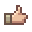#### Zygomorph

##### Spazmatism
Basically yeah. If you know what it is, then I would love to know.

#### Blardow

##### Skeletron Prime
Basically yeah. If you know what it is, then I would love to know.
They are 290 and 294, I don't know what the difference is but you'll need to experiment.

•Zygomorph

#### Sin Costan

##### Eye of Cthulhu
Basically yeah. If you know what it is, then I would love to know.
Sin Costan - [Tutorial]Projectile Guide and Implementation said:
For Vanilla Dust Heat Rays.

Code:
``````{
"displayName": "Test Ray",
"size": [32, 32],
"aiStyle": 0, //I usually just use this for custom AI since it doesn't do anything special
"friendly": true,
"hostile": false,
"tileCollide": true,
"ignoreWater": true,
"magic": true,
"penetrate": -1,
}``````

Code:
``````using System;
using Microsoft.Xna.Framework;
using Microsoft.Xna.Framework.Graphics;

using TAPI;
using Terraria;

namespace COFP.Projectiles
{
public class TestRay : ModProjectile
{
public override void AI()
{
projectile.localAI += 1f; //The timer
if (projectile.localAI > 3f) //The amount of ticks it takes for it to load
{
for (int num562 = 0; num562 < 10; num562++) //Adjust the thickness of the ray; too high a number may cause lag
{
projectile.alpha = 255;
//Dust.newDust(Vector2 position, Size.X, Size.Y, int Dust ID, Velocity.X, Velocity.Y, int alpha (transparency), Color, float scale)
//Size determines where the dust will spawn (at random of course)
//Dust ID's can be found in this handy page http://tconfig.wikia.com/wiki/List_of_Dusts
int num563 = Dust.NewDust(new Vector2(projectile.position.X, projectile.position.Y - projectile.height/4), projectile.width, projectile.height, 60, 0f, 0f, 0, default(Color), 0.75f);
//Vector2(projectile.position.X, projectile.position.Y - projectile.height/4) - (Vector position) makes sure it shoots from the center of where the weapon is pointing
//projectile.width - Sets it to the projectile's size of X in terms of pixels (16 pixels = 1 tile block)
//projectile.height - Sets it to the projectile's size of Y in terms of pixels
//Dust ID - I set it to a red color, but if you want a black ray, use 54
//both of the velocity's set to zero so it won't move around
// alpha - set to zero so color is vibrant for the dust
// default(Color) - set to its default color (does not change color)
// scale - set to 0.75 so the dust isn't humongous for the laser
Main.dust[num563].scale = (float)Main.rand.Next(70, 110) * 0.013f; /*Adjust left and right values to sizes you would like to see for your dust in Main.rand.Next(int LeftEnd, int RightEnd)
Main.dust[num563].velocity *= 0.2f; //sets the velocity of the dust to not move that much (not sure if you would need this or not)
Main.dust[num563].noGravity = true; //Makes sure it doesn't fall on the ground
//You can of course add more dusts to the code, but as I said about the for loop determining thickness, it may cause lag.
}
}
}
}
}``````

Code:
``````using System;
using Microsoft.Xna.Framework;
using Microsoft.Xna.Framework.Graphics;

using TAPI;
using Terraria;

namespace COFP.Projectiles
{
public class TestRay : ModProjectile
{
public override void AI()
{
projectile.localAI += 1f; //The timer
if (projectile.localAI > 3f) //The amount of ticks it takes for it to load
{
for (int num562 = 0; num562 < 10; num562++) //Adjust the thickness of the ray; too high a number may cause lag
{
projectile.alpha = 255;
//Dust.newDust(Vector2 position, Size.X, Size.Y, int Dust ID, Velocity.X, Velocity.Y, int alpha (transparency), Color, float scale)
//Size determines where the dust will spawn (at random of course)
//Dust ID's can be found in this handy page http://tconfig.wikia.com/wiki/List_of_Dusts
int num563 = Dust.NewDust(new Vector2(projectile.position.X, projectile.position.Y - projectile.height/4), projectile.width, projectile.height, 60, 0f, 0f, 0, default(Color), 0.75f);
//Vector2(projectile.position.X, projectile.position.Y - projectile.height/4) - (Vector position) makes sure it shoots from the center of where the weapon is pointing
//projectile.width - Sets it to the projectile's size of X in terms of pixels (16 pixels = 1 tile block)
//projectile.height - Sets it to the projectile's size of Y in terms of pixels
//Dust ID - I set it to a red color, but if you want a black ray, use 54
//both of the velocity's set to zero so it won't move around
// alpha - set to zero so color is vibrant for the dust
// default(Color) - set to its default color (does not change color)
// scale - set to 0.75 so the dust isn't humongous for the laser
Main.dust[num563].scale = (float)Main.rand.Next(70, 110) * 0.013f; /*Adjust left and right values to sizes you would like to see for your dust in Main.rand.Next(int LeftEnd, int RightEnd)
Main.dust[num563].velocity *= 0.2f; //sets the velocity of the dust to not move that much (not sure if you would need this or not)
Main.dust[num563].noGravity = true; //Makes sure it doesn't fall on the ground
//You can of course add more dusts to the code, but as I said about the for loop determining thickness, it may cause lag.
}
}
}
public override bool OnTileCollide(ref Vector2 velocityChange) //Bounce off walls
{
if (projectile.velocity.X != velocityChange.X)
{
projectile.velocity.X = -velocityChange.X;
}
if (projectile.velocity.Y != velocityChange.Y)
{
projectile.velocity.Y = -velocityChange.Y;
}
return false;
}
}
}``````